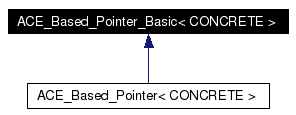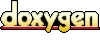# ACE_Based_Pointer_Basic< CONCRETE > Class Template Reference

A proxy that keeps track of the relative offset of a "pointer" from its base address. This class makes it possible to transparently use "pointers" in shared memory as easily as programming with pointers to local memory. In particular, we don't need to ensure that the base addresses of all the pointers are mapped into separate processes at the same absolute memory base address. More...

`#include <Based_Pointer_T.h>`

Inheritance diagram for ACE_Based_Pointer_Basic< CONCRETE >:[legend]
List of all members.

## Public Member Functions

ACE_Based_Pointer_Basic (void)
ACE_Based_Pointer_Basic (CONCRETE *initial)
ACE_Based_Pointer_Basic (const ACE_Based_Pointer_Basic< CONCRETE > &)
Copy constructor.

ACE_Based_Pointer_Basic (const void *base_addr, int o)
void operator= (CONCRETE *from)
Pseudo-assignment operator.

void operator= (const ACE_Based_Pointer_Basic< CONCRETE > &)
Pseudo-assignment operator.

CONCRETE operator * (void) const
Dereference operator.

bool operator< (const ACE_Based_Pointer_Basic< CONCRETE > &) const
Less than operator.

bool operator<= (const ACE_Based_Pointer_Basic< CONCRETE > &) const
Less than or equal operator.

bool operator> (const ACE_Based_Pointer_Basic< CONCRETE > &) const
Greater than operator.

bool operator>= (const ACE_Based_Pointer_Basic< CONCRETE > &) const
Greater than or equal operator.

bool operator== (const ACE_Based_Pointer_Basic< CONCRETE > &) const
Equality operator.

bool operator!= (const ACE_Based_Pointer_Basic< CONCRETE > &) const
Inequality operator.

CONCRETE operator[] (int index) const
Subscript operator.

void operator+= (int index)
Increment operator.

operator CONCRETE * () const
Returns the underlying memory address of the smart pointer.

Returns the underlying memory address of the smart pointer.

void dump (void) const
Dump the state of the object.

## Public Attributes

ACE_ALLOC_HOOK_DECLARE
Declare the dynamic allocation hooks.

## Protected Attributes

ptrdiff_t target_
ptrdiff_t base_offset_
Keep track of our offset from the base pointer.

## Detailed Description

### template<class CONCRETE> class ACE_Based_Pointer_Basic< CONCRETE >

A proxy that keeps track of the relative offset of a "pointer" from its base address. This class makes it possible to transparently use "pointers" in shared memory as easily as programming with pointers to local memory. In particular, we don't need to ensure that the base addresses of all the pointers are mapped into separate processes at the same absolute memory base address.

Definition at line 44 of file Based_Pointer_T.h.

## Constructor & Destructor Documentation

 template ACE_Based_Pointer_Basic< CONCRETE >::ACE_Based_Pointer_Basic ( void )
 This constructor initializes the by asking the Singleton for the base address of the memory region within which it is instantiated. Two results are possible: 1. An has stored a base address/size pair and the new based-pointer instance is located between the base address and the base address + size - 1. In this case, the repository returns the base address. 2. No suitable address/size pair was found. The repository assumes an address in the regular (not mapped) virtual address space of the process and returns 0. In this case, the based-pointer uses its address as an offset to it's base address 0. Definition at line 54 of file Based_Pointer_T.cpp. References ACE_TRACE, and ACE_Based_Pointer_Basic< CONCRETE >::base_offset_. ```00055 : target_ (0), 00056 base_offset_ (0) 00057 { 00058 ACE_TRACE ("ACE_Based_Pointer_Basic::ACE_Based_Pointer_Basic"); 00059 void *base_addr = 0; 00060 00061 // Find the base address associated with our pointer. Note 00062 // that it's ok for to return 0, which simply indicates that 00063 // the address is not in memory-mapped virtual address space. 00064 ACE_BASED_POINTER_REPOSITORY::instance ()->find (this, 00065 base_addr); 00066 this->base_offset_ = (char *) this - (char *) base_addr; 00067 } ```

 template ACE_Based_Pointer_Basic< CONCRETE >::ACE_Based_Pointer_Basic ( CONCRETE * initial )
 Initialize this object using the pointer. This constructor initializes the by asking the Singleton for the base address of the memory region within which it is instantiated. Three results are possible: 1. An has stored a base address/size pair and the new based-pointer instance is located between the base address and the base address + size - 1. In this case, the repository returns the base address. 2. No suitable address/size pair was found. The repository assumes an address in the regular (not mapped) virtual address space of the process and returns 0. In this case, the based-pointer uses its address as an offset to its base address 0. 3. If is 0 then set the value of to -1, which indicates a "NULL" pointer. Definition at line 79 of file Based_Pointer_T.cpp. ```00080 : target_ (0), 00081 base_offset_ (0) 00082 { 00083 ACE_TRACE ("ACE_Based_Pointer_Basic::ACE_Based_Pointer_Basic"); 00084 00085 if (rhs == 0) 00086 // Store a value of that indicate "NULL" pointer. 00087 this->target_ = -1; 00088 else 00089 { 00090 void *base_addr = 0; 00091 00092 // Find the base address associated with the pointer. 00093 // Note that it's ok for to return 0, which simply 00094 // indicates that the address is not in memory-mapped virtual 00095 // address space. 00096 ACE_BASED_POINTER_REPOSITORY::instance ()->find (this, 00097 base_addr); 00098 this->base_offset_ = (char *) this - (char *) base_addr; 00099 this->target_ = ((char *) rhs - (char *) base_addr); 00100 } 00101 } ```

 template ACE_Based_Pointer_Basic< CONCRETE >::ACE_Based_Pointer_Basic ( const ACE_Based_Pointer_Basic< CONCRETE > & )
 Copy constructor. Definition at line 104 of file Based_Pointer_T.cpp. References ACE_ASSERT, and ACE_TRACE. ```00105 { 00106 ACE_TRACE ("ACE_Based_Pointer_Basic::ACE_Based_Pointer_Basic"); 00107 00108 ACE_ASSERT (0); // not implemented. 00109 } ```

 template ACE_Based_Pointer_Basic< CONCRETE >::ACE_Based_Pointer_Basic ( const void * base_addr, int o )
 Constructor for know base address. is only used to resolve overload ambiguity. Definition at line 70 of file Based_Pointer_T.cpp. References ACE_TRACE, and ACE_Based_Pointer_Basic< CONCRETE >::base_offset_. ```00071 : target_ (0), 00072 base_offset_ (0) 00073 { 00074 ACE_TRACE ("ACE_Based_Pointer_Basic::ACE_Based_Pointer_Basic"); 00075 this->base_offset_ = (char *) this - (char *) base_addr; 00076 } ```

## Member Function Documentation

 template ACE_INLINE CONCRETE * ACE_Based_Pointer_Basic< CONCRETE >::addr ( void ) const
 Returns the underlying memory address of the smart pointer. Definition at line 49 of file Based_Pointer_T.inl. ```00050 { 00051 ACE_TRACE ("ACE_Based_Pointer_Basic::addr"); 00052 00053 if (this->target_ == -1) 00054 return 0; 00055 else 00056 return reinterpret_cast (ACE_COMPUTE_BASED_POINTER (this)); 00057 } ```

 template void ACE_Based_Pointer_Basic< CONCRETE >::dump ( void ) const
 Dump the state of the object. Definition at line 25 of file Based_Pointer_T.cpp. ```00026 { 00027 #if defined (ACE_HAS_DUMP) 00028 ACE_TRACE ("ACE_Based_Pointer_Basic::dump"); 00029 00030 ACE_DEBUG ((LM_DEBUG, ACE_BEGIN_DUMP, this)); 00031 ACE_DEBUG ((LM_DEBUG, ACE_LIB_TEXT ("\ntarget_ = %d\n"), this->target_)); 00032 ACE_DEBUG ((LM_DEBUG, ACE_LIB_TEXT ("base_offset_ = %d\n"), this->base_offset_)); 00033 ACE_DEBUG ((LM_DEBUG, ACE_LIB_TEXT ("computed pointer = %x\n"), 00034 (CONCRETE *)(ACE_COMPUTE_BASED_POINTER (this)))); 00035 ACE_DEBUG ((LM_DEBUG, ACE_END_DUMP)); 00036 #endif /* ACE_HAS_DUMP */ 00037 } ```

 template ACE_INLINE CONCRETE ACE_Based_Pointer_Basic< CONCRETE >::operator * ( void ) const
 Dereference operator. Definition at line 42 of file Based_Pointer_T.inl. References ACE_COMPUTE_BASED_POINTER, and ACE_TRACE. ```00043 { 00044 ACE_TRACE ("ACE_Based_Pointer_Basic::operator *"); 00045 return *reinterpret_cast (ACE_COMPUTE_BASED_POINTER (this)); 00046 } ```

 template ACE_INLINE ACE_Based_Pointer_Basic< CONCRETE >::operator CONCRETE * ( ) const
 Returns the underlying memory address of the smart pointer. Definition at line 60 of file Based_Pointer_T.inl. References ACE_TRACE, and ACE_Based_Pointer_Basic< CONCRETE >::addr(). ```00061 { 00062 ACE_TRACE ("ACE_Based_Pointer_Basic::operator CONCRETE *()"); 00063 00064 return this->addr (); 00065 } ```

 template ACE_INLINE bool ACE_Based_Pointer_Basic< CONCRETE >::operator!= ( const ACE_Based_Pointer_Basic< CONCRETE > & ) const
 Inequality operator. Definition at line 91 of file Based_Pointer_T.inl. References ACE_TRACE. ```00092 { 00093 ACE_TRACE ("ACE_Based_Pointer_Basic::operator !="); 00094 return !(*this == rhs); 00095 } ```

 template ACE_INLINE void ACE_Based_Pointer_Basic< CONCRETE >::operator+= ( int index )
 Increment operator. Definition at line 77 of file Based_Pointer_T.inl. References ACE_TRACE, and ACE_Based_Pointer_Basic< CONCRETE >::base_offset_. ```00078 { 00079 ACE_TRACE ("ACE_Based_Pointer_Basic::operator +="); 00080 this->base_offset_ += (index * sizeof (CONCRETE)); 00081 } ```

 template ACE_INLINE bool ACE_Based_Pointer_Basic< CONCRETE >::operator< ( const ACE_Based_Pointer_Basic< CONCRETE > & ) const
 Less than operator. Definition at line 98 of file Based_Pointer_T.inl. References ACE_COMPUTE_BASED_POINTER, and ACE_TRACE. ```00099 { 00100 ACE_TRACE ("ACE_Based_Pointer_Basic::operator <"); 00101 return ACE_COMPUTE_BASED_POINTER (this) < ACE_COMPUTE_BASED_POINTER (&rhs); 00102 } ```

 template ACE_INLINE bool ACE_Based_Pointer_Basic< CONCRETE >::operator<= ( const ACE_Based_Pointer_Basic< CONCRETE > & ) const
 Less than or equal operator. Definition at line 105 of file Based_Pointer_T.inl. References ACE_COMPUTE_BASED_POINTER, and ACE_TRACE. ```00106 { 00107 ACE_TRACE ("ACE_Based_Pointer_Basic::operator <="); 00108 return ACE_COMPUTE_BASED_POINTER (this) <= ACE_COMPUTE_BASED_POINTER (&rhs); 00109 } ```

 template ACE_INLINE void ACE_Based_Pointer_Basic< CONCRETE >::operator= ( const ACE_Based_Pointer_Basic< CONCRETE > & )
 Pseudo-assignment operator. Definition at line 126 of file Based_Pointer_T.inl. References ACE_TRACE, and ACE_Based_Pointer_Basic< CONCRETE >::addr(). ```00127 { 00128 ACE_TRACE ("ACE_Based_Pointer_Basic::operator="); 00129 *this = rhs.addr (); 00130 } ```

 template ACE_INLINE void ACE_Based_Pointer_Basic< CONCRETE >::operator= ( CONCRETE * from )
 Pseudo-assignment operator. Definition at line 18 of file Based_Pointer_T.inl. ```00019 { 00020 ACE_TRACE ("ACE_Based_Pointer_Basic::operator ="); 00021 if (rhs == 0) 00022 // Store a value of that indicate "NULL" pointer. 00023 this->target_ = -1; 00024 else 00025 this->target_ = ((char *) rhs 00026 - ((char *) this - this->base_offset_)); 00027 } ```

 template ACE_INLINE bool ACE_Based_Pointer_Basic< CONCRETE >::operator== ( const ACE_Based_Pointer_Basic< CONCRETE > & ) const
 Equality operator. Definition at line 84 of file Based_Pointer_T.inl. References ACE_COMPUTE_BASED_POINTER, and ACE_TRACE. ```00085 { 00086 ACE_TRACE ("ACE_Based_Pointer_Basic::operator =="); 00087 return ACE_COMPUTE_BASED_POINTER (this) == ACE_COMPUTE_BASED_POINTER (&rhs); 00088 } ```

 template ACE_INLINE bool ACE_Based_Pointer_Basic< CONCRETE >::operator> ( const ACE_Based_Pointer_Basic< CONCRETE > & ) const
 Greater than operator. Definition at line 112 of file Based_Pointer_T.inl. References ACE_COMPUTE_BASED_POINTER, and ACE_TRACE. ```00113 { 00114 ACE_TRACE ("ACE_Based_Pointer_Basic::operator >"); 00115 return ACE_COMPUTE_BASED_POINTER (this) > ACE_COMPUTE_BASED_POINTER (&rhs); 00116 } ```

 template ACE_INLINE bool ACE_Based_Pointer_Basic< CONCRETE >::operator>= ( const ACE_Based_Pointer_Basic< CONCRETE > & ) const
 Greater than or equal operator. Definition at line 119 of file Based_Pointer_T.inl. References ACE_COMPUTE_BASED_POINTER, and ACE_TRACE. ```00120 { 00121 ACE_TRACE ("ACE_Based_Pointer_Basic::operator >="); 00122 return ACE_COMPUTE_BASED_POINTER (this) >= ACE_COMPUTE_BASED_POINTER (&rhs); 00123 } ```

 template ACE_INLINE CONCRETE ACE_Based_Pointer_Basic< CONCRETE >::operator[] ( int index ) const
 Subscript operator. Definition at line 68 of file Based_Pointer_T.inl. References ACE_COMPUTE_BASED_POINTER, and ACE_TRACE. ```00069 { 00070 ACE_TRACE ("ACE_Based_Pointer_Basic::operator []"); 00071 CONCRETE *c = 00072 reinterpret_cast (ACE_COMPUTE_BASED_POINTER (this)); 00073 return c[index]; 00074 } ```

## Member Data Documentation

 template ACE_Based_Pointer_Basic< CONCRETE >::ACE_ALLOC_HOOK_DECLARE
 Declare the dynamic allocation hooks. Definition at line 136 of file Based_Pointer_T.h.

 template ptrdiff_t ACE_Based_Pointer_Basic< CONCRETE >::base_offset_` [protected]`
 Keep track of our offset from the base pointer. Definition at line 145 of file Based_Pointer_T.h.

 template ptrdiff_t ACE_Based_Pointer_Basic< CONCRETE >::target_` [protected]`
 Definition at line 142 of file Based_Pointer_T.h.

The documentation for this class was generated from the following files:
Generated on Thu Nov 9 11:20:18 2006 for ACE by1.3.6